# Access Algebra 1A (#7912080)

This document was generated on CPALMS - www.cpalms.org
You are not viewing the current course, please click the current year’s tab.

#### Course Standards

Name Description
MA.912.AR.1.1: Identify and interpret parts of an equation or expression that represent a quantity in terms of a mathematical or real-world context, including viewing one or more of its parts as a single entity.
 Clarifications:Clarification 1: Parts of an expression include factors, terms, constants, coefficients and variables. Clarification 2: Within the Mathematics for Data and Financial Literacy course, problem types focus on money and business.

 Examples:Algebra 1 Example: Derrick is using the formula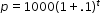to make a prediction about the camel population in Australia. He identifies the growth factor as (1+.1), or 1.1, and states that the camel population will grow at an annual rate of 10% per year.Example: The expression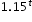can be rewritten as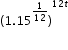which is approximately equivalent to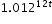. This latter expression reveals the approximate equivalent monthly interest rate of 1.2% if the annual rate is 15%.
##### Related Access Points
 Name Description MA.912.AR.1.AP.1: Identify a part(s) of an equation or expression and explain the meaning within the context of a problem.

MA.912.AR.1.2: Rearrange equations or formulas to isolate a quantity of interest.
 Clarifications:Clarification 1: Instruction includes using formulas for temperature, perimeter, area and volume; using equations for linear (standard, slope-intercept and point-slope forms) and quadratic (standard, factored and vertex forms) functions. Clarification 2: Within the Mathematics for Data and Financial Literacy course, problem types focus on money and business.

 Examples:Algebra 1 Example: The Ideal Gas Law PV = nRT can be rearranged as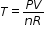to isolate temperature as the quantity of interest. Example: Given the Compound Interest formula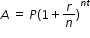, solve for P. Mathematics for Data and Financial Literacy Honors Example: Given the Compound Interest formula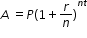, solve for t.
##### Related Access Points
 Name Description MA.912.AR.1.AP.2: Rearrange an equation or a formula for a specific variable.

MA.912.AR.2.1: Given a real-world context, write and solve one-variable multi-step linear equations.
##### Related Access Points
 Name Description MA.912.AR.2.AP.1: Given an equation in a real-world context, solve one-variable multi-step linear equations.

MA.912.AR.2.2: Write a linear two-variable equation to represent the relationship between two quantities from a graph, a written description or a table of values within a mathematical or real-world context.
 Clarifications:Clarification 1: Instruction includes the use of standard form, slope-intercept form and point-slope form, and the conversion between these forms.
##### Related Access Points
 Name Description MA.912.AR.2.AP.2: Select a linear two-variable equation to represent relationships between quantities from a graph, a written description or a table of values within a mathematical or real-world context.

MA.912.AR.2.3: Write a linear two-variable equation for a line that is parallel or perpendicular to a given line and goes through a given point.
 Clarifications:Clarification 1: Instruction focuses on recognizing that perpendicular lines have slopes that when multiplied result in -1 and that parallel lines have slopes that are the same. Clarification 2: Instruction includes representing a line with a pair of points on the coordinate plane or with an equation. Clarification 3: Problems include cases where one variable has a coefficient of zero.
##### Related Access Points
 Name Description MA.912.AR.2.AP.3: Select a linear two-variable equation in slope intercept form for a line that is parallel or perpendicular to a given line and goes through a given point.

MA.912.AR.2.4: Given a table, equation or written description of a linear function, graph that function, and determine and interpret its key features.
 Clarifications:Clarification 1: Key features are limited to domain, range, intercepts and rate of change. Clarification 2: Instruction includes the use of standard form, slope-intercept form and point-slope form.Clarification 3: Instruction includes cases where one variable has a coefficient of zero.Clarification 4: Instruction includes representing the domain and range with inequality notation, interval notation or set-builder notation.Clarification 5: Within the Algebra 1 course, notations for domain and range are limited to inequality and set-builder notations.
##### Related Access Points
 Name Description MA.912.AR.2.AP.4: Given a table, equation or written description of a linear function, select a graph of that function and determine at least two key features (can include domain, range, y-intercept or slope).

MA.912.AR.2.5: Solve and graph mathematical and real-world problems that are modeled with linear functions. Interpret key features and determine constraints in terms of the context.
 Clarifications:Clarification 1: Key features are limited to domain, range, intercepts and rate of change.Clarification 2: Instruction includes the use of standard form, slope-intercept form and point-slope form.Clarification 3: Instruction includes representing the domain, range and constraints with inequality notation, interval notation or set-builder notation.Clarification 4: Within the Algebra 1 course, notations for domain, range and constraints are limited to inequality and set-builder.Clarification 5: Within the Mathematics for Data and Financial Literacy course, problem types focus on money and business.

 Examples:Algebra 1 Example: Lizzy’s mother uses the function C(p)=450+7.75p, where C(p) represents the total cost of a rental space and p is the number of people attending, to help budget Lizzy’s 16th birthday party. Lizzy’s mom wants to spend no more than \$850 for the party. Graph the function in terms of the context.
##### Related Access Points
 Name Description MA.912.AR.2.AP.5: Given a mathematical and/or real-world problem that is modeled with linear functions, solve the mathematical problem, or select the graph using key features (in terms of context) that represents this model.

MA.912.AR.2.6: Given a mathematical or real-world context, write and solve one-variable linear inequalities, including compound inequalities. Represent solutions algebraically or graphically.
 Examples:Algebra 1 Example: The compound inequality 2x≤5x+1<4 is equivalent to -1≤3x and 5x<3, which is equivalent to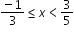.
##### Related Access Points
 Name Description MA.912.AR.2.AP.6: Given a mathematical and/or real-world context, select a one-variable linear inequality that represents the solution algebraically or graphically.

MA.912.AR.2.7: Write two-variable linear inequalities to represent relationships between quantities from a graph or a written description within a mathematical or real-world context.
 Clarifications:Clarification 1: Instruction includes the use of standard form, slope-intercept form and point-slope form and any inequality symbol can be represented. Clarification 2: Instruction includes cases where one variable has a coefficient of zero.
##### Related Access Points
 Name Description MA.912.AR.2.AP.7: Select a two-variable linear inequality to represent relationships between quantities from a graph.

MA.912.AR.2.8: Given a mathematical or real-world context, graph the solution set to a two-variable linear inequality.
 Clarifications:Clarification 1: Instruction includes the use of standard form, slope-intercept form and point-slope form and any inequality symbol can be represented. Clarification 2: Instruction includes cases where one variable has a coefficient of zero.
##### Related Access Points
 Name Description MA.912.AR.2.AP.8: Given a two-variable linear inequality, select a graph that represents the solution.

MA.912.AR.4.1: Given a mathematical or real-world context, write and solve one-variable absolute value equations.
##### Related Access Points
 Name Description MA.912.AR.4.AP.1: Solve a one variable absolute value equation.

MA.912.AR.4.3: Given a table, equation or written description of an absolute value function, graph that function and determine its key features.
 Clarifications:Clarification 1: Key features are limited to domain; range; intercepts; intervals where the function is increasing, decreasing, positive or negative; vertex; end behavior and symmetry. Clarification 2: Instruction includes representing the domain and range with inequality notation, interval notation or set-builder notation.Clarification 3: Within the Algebra 1 course, notations for domain and range are limited to inequality and set-builder.
##### Related Access Points
 Name Description MA.912.AR.4.AP.3: Given a table, equation or written description of an absolute value function, select the graph that represents the function.

MA.912.AR.9.1: Given a mathematical or real-world context, write and solve a system of two-variable linear equations algebraically or graphically.
 Clarifications:Clarification 1: Within this benchmark, the expectation is to solve systems using elimination, substitution and graphing.Clarification 2: Within the Algebra 1 course, the system is limited to two equations.
##### Related Access Points
 Name Description MA.912.AR.9.AP.1: Given an algebraic or graphical system of two-variable linear equations, select the solution to the system of equations.

MA.912.AR.9.4: Graph the solution set of a system of two-variable linear inequalities.
 Clarifications:Clarification 1: Instruction includes cases where one variable has a coefficient of zero. Clarification 2: Within the Algebra 1 course, the system is limited to two inequalities.
##### Related Access Points
 Name Description MA.912.AR.9.AP.4: Select the graph of the solution set of a system of two-variable linear inequalities.

MA.912.AR.9.6: Given a real-world context, represent constraints as systems of linear equations or inequalities. Interpret solutions to problems as viable or non-viable options.
 Clarifications:Clarification 1: Instruction focuses on analyzing a given function that models a real-world situation and writing constraints that are represented as linear equations or linear inequalities.
##### Related Access Points
 Name Description MA.912.AR.9.AP.6: Given a real-world context, as systems of linear equations or inequalities with identified constraints, select a solution as a viable or non-viable option.

MA.912.DP.1.3: Explain the difference between correlation and causation in the contexts of both numerical and categorical data.
 Examples:Algebra 1 Example: There is a strong positive correlation between the number of Nobel prizes won by country and the per capita chocolate consumption by country. Does this mean that increased chocolate consumption in America will increase the United States of America’s chances of a Nobel prize winner?
##### Related Access Points
 Name Description MA.912.DP.1.AP.3: Identify whether the data are explained by correlation or causation in the contexts of both numerical and categorical data.

MA.912.DP.2.4: Fit a linear function to bivariate numerical data that suggests a linear association and interpret the slope and y-intercept of the model. Use the model to solve real-world problems in terms of the context of the data.
 Clarifications:Clarification 1: Instruction includes fitting a linear function both informally and formally with the use of technology.Clarification 2: Problems include making a prediction or extrapolation, inside and outside the range of the data, based on the equation of the line of fit.
##### Related Access Points
 Name Description MA.912.DP.2.AP.4: Fit a linear function to bivariate numerical data that suggest a linear association and interpret the slope and y-intercept of the model.

MA.912.DP.2.6: Given a scatter plot with a line of fit and residuals, determine the strength and direction of the correlation. Interpret strength and direction within a real-world context.
 Clarifications:Clarification 1: Instruction focuses on determining the direction by analyzing the slope and informally determining the strength by analyzing the residuals.
##### Related Access Points
 Name Description MA.912.DP.2.AP.6: Given a scatter plot with a line of fit and residuals, determine the strength and direction of the correlation. Interpret strength and direction within a real-world context.

MA.912.F.1.1: Given an equation or graph that defines a function, determine the function type. Given an input-output table, determine a function type that could represent it.
 Clarifications:Clarification 1: Within the Algebra 1 course, functions represented as tables are limited to linear, quadratic and exponential. Clarification 2: Within the Algebra 1 course, functions represented as equations or graphs are limited to vertical or horizontal translations or reflections over the x-axis of the following parent functions: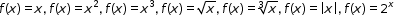and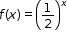.
##### Related Access Points
 Name Description MA.912.F.1.AP.1a: Given an equation or graph that defines a function, identify the function type as either linear, quadratic, or exponential. MA.912.F.1.AP.1b: Given an input-output table with an accompanying graph, determine a function type, either linear, quadratic, or exponential that could represent it.

MA.912.F.1.2: Given a function represented in function notation, evaluate the function for an input in its domain. For a real-world context, interpret the output.
 Clarifications:Clarification 1: Problems include simple functions in two-variables, such as f(x,y)=3x-2y. Clarification 2: Within the Algebra 1 course, functions are limited to one-variable such as f(x)=3x.

 Examples:Algebra 1 Example: The function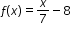models Alicia’s position in miles relative to a water stand x minutes into a marathon. Evaluate and interpret for a quarter of an hour into the race.
##### Related Access Points
 Name Description MA.912.F.1.AP.2: Given a function represented in function notation, evaluate the function for an input in its domain.

MA.912.F.1.3: Calculate and interpret the average rate of change of a real-world situation represented graphically, algebraically or in a table over a specified interval.
 Clarifications:Clarification 1: Instruction includes making the connection to determining the slope of a particular line segment.
##### Related Access Points
 Name Description MA.912.F.1.AP.3: Given a real-world situation represented graphically or algebraically, identify the rate of change as positive, negative, zero or undefined.

MA.912.F.1.5: Compare key features of linear functions each represented algebraically, graphically, in tables or written descriptions.
 Clarifications:Clarification 1: Key features are limited to domain; range; intercepts; slope and end behavior.
##### Related Access Points
 Name Description MA.912.F.1.AP.5: Identify key features of linear and quadratic functions each represented in the same way algebraically or graphically (key features are limited to domain; range; intercepts; intervals where the function is increasing, decreasing, positive or negative; end behavior).

MA.912.F.1.8: Determine whether a linear, quadratic or exponential function best models a given real-world situation.
 Clarifications:Clarification 1: Instruction includes recognizing that linear functions model situations in which a quantity changes by a constant amount per unit interval; that quadratic functions model situations in which a quantity increases to a maximum, then begins to decrease or a quantity decreases to a minimum, then begins to increase; and that exponential functions model situations in which a quantity grows or decays by a constant percent per unit interval. Clarification 2: Within this benchmark, the expectation is to identify the type of function from a written description or table.
##### Related Access Points
 Name Description MA.912.F.1.AP.8: Select whether a linear or quadratic function best models a given real-world situation.

MA.912.F.2.1: Identify the effect on the graph or table of a given function after replacing f(x) by f(x)+k,kf(x), f(kx) and f(x+k) for specific values of k.
 Clarifications:Clarification 1: Within the Algebra 1 course, functions are limited to linear, quadratic and absolute value.Clarification 2: Instruction focuses on including positive and negative values for k.
##### Related Access Points
 Name Description MA.912.F.2.AP.1: Select the effect (up, down, left, or right) on the graph of a given function after replacing f(x) by f(x) + k and f(x + k) for specific values of k.

MA.912.FL.3.2: Solve real-world problems involving simple, compound and continuously compounded interest.
 Clarifications:Clarification 1: Within the Algebra 1 course, interest is limited to simple and compound.

 Examples:Example: Find the amount of money on deposit at the end of 5 years if you started with \$500 and it was compounded quarterly at 6% interest per year. Example: Joe won \$25,000 on a lottery scratch-off ticket. How many years will it take at 6% interest compounded yearly for his money to double?
##### Related Access Points
 Name Description MA.912.FL.3.AP.2: Solve real-world problems involving simple and compound interest.

MA.912.FL.3.4: Explain the relationship between simple interest and linear growth. Explain the relationship between compound interest and exponential growth and the relationship between continuously compounded interest and exponential growth.
 Clarifications:Clarification 1: Within the Algebra 1 course, exponential growth is limited to compound interest.
##### Related Access Points
 Name Description MA.912.FL.3.AP.4: Identify the relationship between simple interest and linear growth. Identify the relationship between compound interest and exponential growth.

MA.K12.MTR.1.1: Actively participate in effortful learning both individually and collectively.

Mathematicians who participate in effortful learning both individually and with others:

• Analyze the problem in a way that makes sense given the task.
• Build perseverance by modifying methods as needed while solving a challenging task.
• Stay engaged and maintain a positive mindset when working to solve tasks.
• Help and support each other when attempting a new method or approach.

 Clarifications:Teachers who encourage students to participate actively in effortful learning both individually and with others: Cultivate a community of growth mindset learners.  Foster perseverance in students by choosing tasks that are challenging. Develop students’ ability to analyze and problem solve. Recognize students’ effort when solving challenging problems.
MA.K12.MTR.2.1: Demonstrate understanding by representing problems in multiple ways.

Mathematicians who demonstrate understanding by representing problems in multiple ways:

• Build understanding through modeling and using manipulatives.
• Represent solutions to problems in multiple ways using objects, drawings, tables, graphs and equations.
• Progress from modeling problems with objects and drawings to using algorithms and equations.
• Express connections between concepts and representations.
• Choose a representation based on the given context or purpose.
 Clarifications:Teachers who encourage students to demonstrate understanding by representing problems in multiple ways: Help students make connections between concepts and representations.Provide opportunities for students to use manipulatives when investigating concepts.Guide students from concrete to pictorial to abstract representations as understanding progresses.Show students that various representations can have different purposes and can be useful in different situations.
MA.K12.MTR.3.1: Complete tasks with mathematical fluency.

Mathematicians who complete tasks with mathematical fluency:

• Select efficient and appropriate methods for solving problems within the given context.
• Maintain flexibility and accuracy while performing procedures and mental calculations.
• Complete tasks accurately and with confidence.
• Adapt procedures to apply them to a new context.
• Use feedback to improve efficiency when performing calculations.
 Clarifications:Teachers who encourage students to complete tasks with mathematical fluency:Provide students with the flexibility to solve problems by selecting a procedure that allows them to solve efficiently and accurately.Offer multiple opportunities for students to practice efficient and generalizable methods.Provide opportunities for students to reflect on the method they used and determine if a more efficient method could have been used.
MA.K12.MTR.4.1: Engage in discussions that reflect on the mathematical thinking of self and others.

Mathematicians who engage in discussions that reflect on the mathematical thinking of self and others:

• Communicate mathematical ideas, vocabulary and methods effectively.
• Analyze the mathematical thinking of others.
• Compare the efficiency of a method to those expressed by others.
• Recognize errors and suggest how to correctly solve the task.
• Justify results by explaining methods and processes.
• Construct possible arguments based on evidence.
 Clarifications:Teachers who encourage students to engage in discussions that reflect on the mathematical thinking of self and others:Establish a culture in which students ask questions of the teacher and their peers, and error is an opportunity for learning.Create opportunities for students to discuss their thinking with peers.Select, sequence and present student work to advance and deepen understanding of correct and increasingly efficient methods.Develop students’ ability to justify methods and compare their responses to the responses of their peers.
MA.K12.MTR.5.1: Use patterns and structure to help understand and connect mathematical concepts.

Mathematicians who use patterns and structure to help understand and connect mathematical concepts:

• Focus on relevant details within a problem.
• Create plans and procedures to logically order events, steps or ideas to solve problems.
• Decompose a complex problem into manageable parts.
• Relate previously learned concepts to new concepts.
• Look for similarities among problems.
• Connect solutions of problems to more complicated large-scale situations.
 Clarifications:Teachers who encourage students to use patterns and structure to help understand and connect mathematical concepts:Help students recognize the patterns in the world around them and connect these patterns to mathematical concepts.Support students to develop generalizations based on the similarities found among problems.Provide opportunities for students to create plans and procedures to solve problems.Develop students’ ability to construct relationships between their current understanding and more sophisticated ways of thinking.
MA.K12.MTR.6.1: Assess the reasonableness of solutions.

Mathematicians who assess the reasonableness of solutions:

• Estimate to discover possible solutions.
• Use benchmark quantities to determine if a solution makes sense.
• Check calculations when solving problems.
• Verify possible solutions by explaining the methods used.
• Evaluate results based on the given context.
 Clarifications:Teachers who encourage students to assess the reasonableness of solutions:Have students estimate or predict solutions prior to solving.Prompt students to continually ask, “Does this solution make sense? How do you know?”Reinforce that students check their work as they progress within and after a task.Strengthen students’ ability to verify solutions through justifications.
MA.K12.MTR.7.1: Apply mathematics to real-world contexts.

Mathematicians who apply mathematics to real-world contexts:

• Connect mathematical concepts to everyday experiences.
• Use models and methods to understand, represent and solve problems.
• Perform investigations to gather data or determine if a method is appropriate. • Redesign models and methods to improve accuracy or efficiency.
 Clarifications:Teachers who encourage students to apply mathematics to real-world contexts:Provide opportunities for students to create models, both concrete and abstract, and perform investigations.Challenge students to question the accuracy of their models and methods.Support students as they validate conclusions by comparing them to the given situation.Indicate how various concepts can be applied to other disciplines.
ELA.K12.EE.1.1: Cite evidence to explain and justify reasoning.
 Clarifications:K-1 Students include textual evidence in their oral communication with guidance and support from adults. The evidence can consist of details from the text without naming the text. During 1st grade, students learn how to incorporate the evidence in their writing.2-3 Students include relevant textual evidence in their written and oral communication. Students should name the text when they refer to it. In 3rd grade, students should use a combination of direct and indirect citations.4-5 Students continue with previous skills and reference comments made by speakers and peers. Students cite texts that they’ve directly quoted, paraphrased, or used for information. When writing, students will use the form of citation dictated by the instructor or the style guide referenced by the instructor. 6-8 Students continue with previous skills and use a style guide to create a proper citation.9-12 Students continue with previous skills and should be aware of existing style guides and the ways in which they differ.
 Clarifications:See Text Complexity for grade-level complexity bands and a text complexity rubric.
ELA.K12.EE.3.1: Make inferences to support comprehension.
 Clarifications:Students will make inferences before the words infer or inference are introduced. Kindergarten students will answer questions like “Why is the girl smiling?” or make predictions about what will happen based on the title page. Students will use the terms and apply them in 2nd grade and beyond.
ELA.K12.EE.4.1: Use appropriate collaborative techniques and active listening skills when engaging in discussions in a variety of situations.
 Clarifications:In kindergarten, students learn to listen to one another respectfully.In grades 1-2, students build upon these skills by justifying what they are thinking. For example: “I think ________ because _______.” The collaborative conversations are becoming academic conversations.In grades 3-12, students engage in academic conversations discussing claims and justifying their reasoning, refining and applying skills. Students build on ideas, propel the conversation, and support claims and counterclaims with evidence.
ELA.K12.EE.5.1: Use the accepted rules governing a specific format to create quality work.
 Clarifications:Students will incorporate skills learned into work products to produce quality work. For students to incorporate these skills appropriately, they must receive instruction. A 3rd grade student creating a poster board display must have instruction in how to effectively present information to do quality work.
ELA.K12.EE.6.1: Use appropriate voice and tone when speaking or writing.
 Clarifications:In kindergarten and 1st grade, students learn the difference between formal and informal language. For example, the way we talk to our friends differs from the way we speak to adults. In 2nd grade and beyond, students practice appropriate social and academic language to discuss texts.
ELD.K12.ELL.MA.1: English language learners communicate information, ideas and concepts necessary for academic success in the content area of Mathematics.
ELD.K12.ELL.SI.1: English language learners communicate for social and instructional purposes within the school setting.

## General Course Information and Notes

### VERSION DESCRIPTION

Access Courses:

Access courses are for students with the most significant cognitive disabilities. Access courses are designed to provide students access to grade-level general curriculum. Access points are alternate academic achievement standards included in access courses that target the salient content of Florida’s standards. Access points are intentionally designed to academically challenge students with the most significant cognitive disabilities.

### GENERAL NOTES

English Language Development ELD Standards Special Notes Section:

Teachers are required to provide listening, speaking, reading and writing instruction that allows English language learners (ELL) to communicate information, ideas and concepts for academic success in the content area of Language Arts. For the given level of English language proficiency and with visual, graphic, or interactive support, students will interact with grade level words, expressions, sentences and discourse to process or produce language necessary for academic success. The ELD standard should specify a relevant content area concept or topic of study chosen by curriculum developers and teachers which maximizes an ELL's need for communication and social skills. To access an ELL supporting document which delineates performance definitions and descriptors, please click on the following link: https://cpalmsmediaprod.blob.core.windows.net/uploads/docs/standards/eld/la.pdf.

### General Information

 Course Number: 7912080 Course Path: Section: Exceptional Student Education > Grade Group: Senior High and Adult > Subject: Academics - Subject Areas > Abbreviated Title: ACCESS ALGEBRA 1A Number of Credits: Course may be taken for up to two credits Course Attributes: Class Size Core Required Florida Standards Course Course Type: Core Academic Course Course Status: Course Approved Graduation Requirement: Mathematics